# Pointers to Pointers in C Programming

We have seen how to declare, initialize and use a pointer variable. We have understood that pointer is also a variable, which stores the address of another variable. That means even though it is a pointer, it is also a variable, and has memory address. Hence we can have another pointer to hold the address of this pointer variable. This kind of pointers are called pointer to a pointer. Below diagram shows intPtrXX is a pointer to a pointer.

```int intX; // a integer variable
int *intPtrX; // a integer pointer
int **intPtrXX; // a integer pointer to a pointer```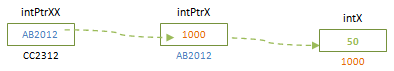We can note that a pointer to a pointer has double ‘*’ before its name. This is because, it has to indicate compiler that it is a pointer which holds the address of another pointer. When compiler evaluates double pointer, it knows that it has to evaluate the address two times, so that it gets the actual value that it is pointing to. In above case, compiler evaluates value in intPtrXX, AB2012 as address and it knows that it is an address of another pointer. Hence it goes to that memory location to see another address, 1000 and evaluates it to find the actual value stored at 1000 – which is 50.

Below program shows how a pointer to a pointer can be declared, initialized and accessed. It shows how each value and address of pointers changes. The syntax intPtrXX = &intPtrX; indicates that intPtrXX holds the address of pointer intPtrX, which makes it a pointer to a pointer. When we change the value of intPtrXX by assigning it 200, it actually changes the value of integer variable, intX. Hence we can see that the pointer variable intPtrX also has changed its value to 200.

```#include <stdio.h>

int main()
{
int intX; // a integer variable
int *intPtrX; // a integer pointer
int **intPtrXX; // a integer pointer to a pointer

intPtrX = &intX; // Assign the address of intX to pointer intPtrX
intPtrXX = &intPtrX; // Assign the address of intPtrX to intPtrXX

intX = 50; // Assign the value to integer variable

// Values in different variables are accessed as below
printf("Value of integer variable is : %d\n", intX); // Value of integer variable is: 50
printf("Value of pointer variable is : %d\n", *intPtrX); // Value of pointer variable is: 50
printf("Value of pointed by pointer to a pointer is : %d\n", **intPtrXX); // Value of pointed by pointer to a pointer is: 50

// Addresses of variables and addresses they hold are accesses as below
printf("Address of integer variable is : %x\n", &intX); //1000
printf("Address of pointer variable is : %x\n", &intPtrX); // AB2012
printf("Address pointed by a pointer variable is : %x\n", intPtrX); //1000
printf("Address of pointed by pointer to a pointer is : %x\n", &intPtrXX); // CC2312
printf("Address pointed by a pointer to a pointer is : %x\n", intPtrXX); // AB2012

**intPtrXX = 200;

printf("\nAfter changing the pointer value\n");
printf("---------------------------------------\n");
// Displays new value 200
printf("Value of integer variable is : %d\n", intX); // Value of integer variable is: 200
printf("Value of pointer variable is : %d\n", *intPtrX); // Value of pointer variable is: 200
printf("Value of pointed by pointer to a pointer is : %d\n", **intPtrXX); // Value of pointed by pointer to a pointer is: 200

printf("Address of integer variable is : %x\n", &intX); //1000
printf("Address of pointer variable is : %x\n", &intPtrX); // AB2012
printf("Address pointed by a pointer variable is : %x\n", intPtrX); //1000
printf("Address of pointed by pointer to a pointer is : %x\n", &intPtrXX); // CC2312
printf("Address pointed by a pointer to a pointer is : %x\n", intPtrXX); // AB2012

return 0;

}```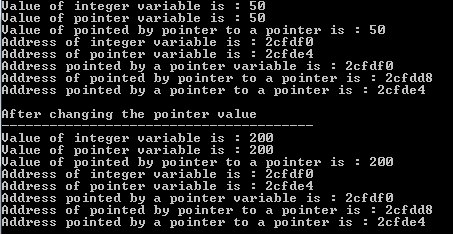In order to understand pointers to pointers, let us consider a two dimensional array, intArr  . What does this array infer? It has 3 rows with 2 columns. This means there are 3 similar arrays with two elements each, stored in the contiguous memory locations as shown in the second diagram below.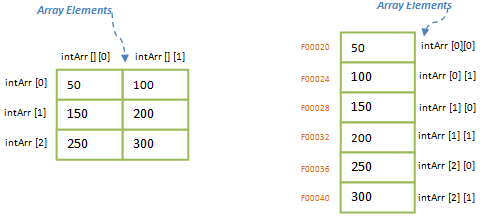As we stated earlier that multidimensional array is multiple arrays with same number of elements, above array can be re-written as (intArr )  (please note this notation is for understanding purpose) as shown in first diagram above. This means we have 3 arrays with 2 columns each. But the single dimension array intArr  can be replaced with a pointer variable, i.e.;

`int *intPtrRow ;`

Here pointer intPtrRow points to the beginning element of the two dimensional array, intArr  . Since intPtrRow is a pointer to an array of 3 rows, as we increment this pointer it will point to next row element of two-dimensional array. That means, when we increment intPtrRow, it will point to intArr , intArr  etc, which are the rows of 2-dimensional array. Hence it achieves two-dimensional array.

intArr   → intArr   → *intPtrRow  → intPtrArr is a pointer to an array of 3 elements, which in turn an array of two elements (check diagram below).

i.e.; intPtrRow = &intArr  → intArr  

Hence as we increment it points to elements of intArr , which is the beginning of each row of two-dimensional array.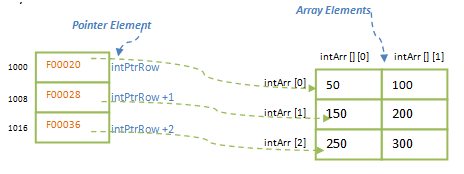Now if we observe the pointer that we defined above, * intPtrRow , is an array again. Can we have a pointer to this array? Yes, we can assign a pointer to this array too. This new pointer will be pointing each element in the array *intPtrRow . That means using this second pointer we can even access the columns of 2-dimensional array. As we increment this pointer, it will point to the columns of 2D array. Below diagram will make this clearer.

`int **intPtrCol = &intPtrRow;`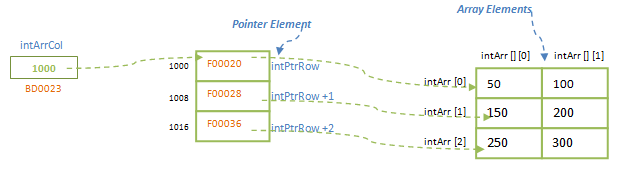One may wonder how incrementing the second pointer, intPtrCol will point to the columns of the array. Since the first pointer is an array, when we allocate memory to the pointer intPtrRow, memory for two integer element will be allocated, i.e.; as simple as allocating memory for array of two integer element. Hence above pointer will have 8 bytes of memory allocated (can see them in diagram above). Hence we increment the pointer, intPtrRow, it will increment the memory blocks by 8, and it will point to next row of 2-dimensional array.

Now another pointer is defined on this pointer array. But new pointer is a simple integer pointer (not array). Hence only 4 bytes of memory is allocated to it. Hence when we increment the pointer, intPtrCol, 4bytes of memory blocks will be moved further, which is a second column of 2-dimensional array. Closely observe how memory blocks are incremented in each pointer, when they are incremented.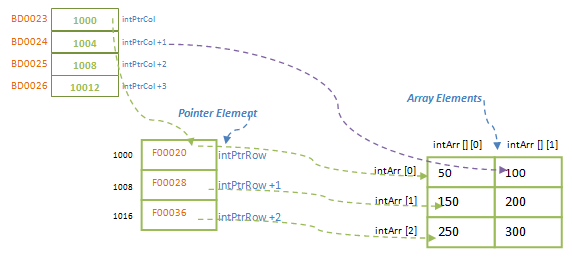In memory, 2-dimensional array is distributed in contiguous memory locations. Hence when we define pointers to its rows and columns, it will look like below. When intPtrCol is having address F00020, it points to first element of intPtrRow, which is in turn first element of 2D array. In below diagram, you may see that when intPtrCol is incremented by 1, it has the address 1004; but it is pointing to the address F00024. How is this possible? This is because of the same reason explained above – since intPtrCol is a pointer to a array pointer, when it is incremented by one, its address is updated to 1004. But it will also increment the address stored in the array pointer, intPtrRow by 4 which is F00024 – address of second column of the array. Hence even though the address pointed by intPtrCol is 1004, it will point to the address F00024 pointed by the array pointer at 1004.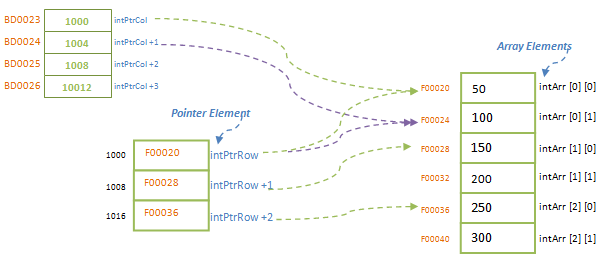In above case, we have used two pointer variables to point to a 2D array. This kind of pointer to a pointer will be represented as **intPtr, where double ‘*’ before the pointer name to indicate pointer to a pointer.  When a pointer is a double pointer or pointer to a pointer, then it works in the same way as explained above. We can understand this concept of double pointers by evaluating 2D array as below using array and pointers:

intArr [i] [j] = *(intArr +i) [j] = *(*(intArr +i) +j) = **intArr → 2D array is a pointer to a pointer.

Program below shows how a 2D array works when pointer is used to point them.

```#include <stdio.h>
#define ROWS 3
#define COLS 2

int main()
{
int   intArr[ROWS][COLS]; // 2D array declaration

int i, j;

// Requests users to enter the value for elements of 2D array
for (i = 0; i< ROWS; i++) {
for (j = 0; j< COLS; j++) {
printf("Enter the value for array intArr[%d][%d]:", i, j);
scanf("%d", &intArr[i][j]);
}
}

printf("\nTwo dimensional array in Matrix Form\n");
for (i = 0; i< ROWS; i++) {
for (j = 0; j< COLS; j++) {
printf("%d\t", *(*(intArr + i) + j)); // array is used as pointer to a pointer
}
printf("\n");
}
return 0;
}```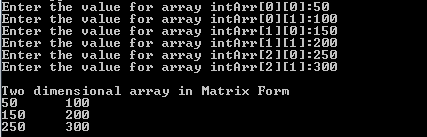Translate »